HomeBlog

# Discussion on the influencing factors of clock in FPGA design

Summary

The clock is the most important and special signal in the entire circuit. The movement of most of the devices in the system is performed on the edge of the clock. This requires that the delay of the clock signal is very small, otherwise it may cause an error in the timing logic. Therefore, it is very important for the design of FPGA to determine the factors of system clock and the delay of clock to ensure the stability of design.

 Core Clock in FPGA design Purpose Determining the influencing factors of clock to ensure the stability of design English name Field Programmable Gate Array Category Digital electronic circuit Function Creating digital circuits Feature Totally up to the designer to create a bit file

Catalogs

 Catalogs Ⅰ. What is Setup time and Hold time Ⅲ. Analyzing with the help of timing diagram 3. The composition of the state machine 1. Synchronization between single bits and each pulse transmitted has at least 1 cycle width 1. Setup time Ⅳ. How to increase the clock working frequency Ⅴ. An example showing a good method for state machine design 2. The input pulse could be less than a synchronous circuit under a clock cycle width 2. Hold time 1. Changing the line type for circuit wiring Ⅵ. The introduction of state machine Ⅱ. A basic model of synchronous design using a single clock 2. Splitting the combinational logic Ⅶ. What we should pay attention when designing the clock in FPGA

Introduction

Ⅰ. What is Setup time and Hold time

The clock is the most important and special signal in the entire circuit. The movement of most of the devices in the system is performed on the edge of the clock. This requires that the delay of the clock signal is very small, otherwise it may cause an error in the timing logic. Therefore, it is very important for the design of FPGA to determine the factors of the system clock and the delay of the clock to ensure the stability of the design.

Learn how a clock drives all sequential logic in FPGA, from Flip-Flops to Block RAMs;

The clock tells you how fast you can run your FPGA;

This video demonstrates how to properly deal with multiple clock domains inside your design.

1. Setup time

Setup time(Tsu) is defined as the minimum amount of time before the clock's active edge that the data must be stable for it to be latched correctly. Any violation may cause incorrect data to be captured, which is known as setup violation.

2. Hold time

Hold time(Thd) is defined as the minimum amount of time after the clock's active edge during which data must be stable. Violation in this case may cause incorrect data to be latched, which is known as a hold violation. Note that setup and hold time is measured with respect to the active clock edge only.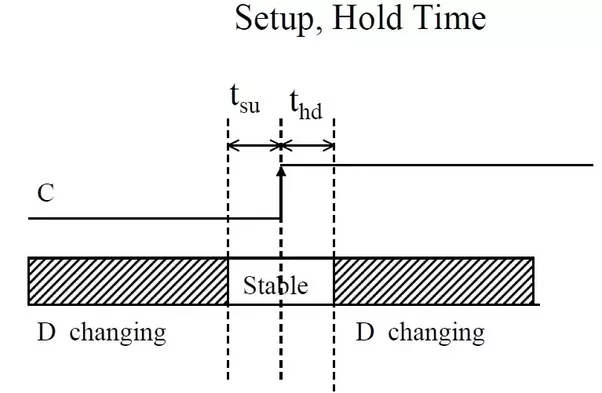Figure 1 Shows setup time and hold timeFigure 2 If data will change in tsu then it will cause setup violation and if data will change in thd then it will cause hold violation

Dtail

Ⅱ. A basic model of synchronous design using a single clock

In the same module of FPGA design, it often contains the combinational logic and the sequential logic. In order to guarantee the data in this logic interface can be processed steadily, then figuring out the concept of setup time and hold time is very important. Then we could be able to think about this following question: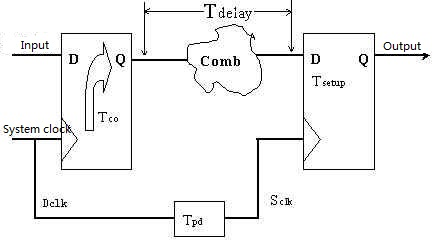Figure 3 Shows a basic model of synchronous design using a single clock

Tco: Delay of the data output of the trigger;

Tdelay: Delay of the combinational logic;

Tsetup: The trigger's setup time;

Tpd: Delay of the clock (negligible).

T: clock cycle

T3: D2 setup time

T4: D2 hold time

If the first trigger D1 has a maximum setup time of T1max and a minimum of T1min, the combinational logic has a maximum delay of T2max and a minimum of T2min. The question is what conditions setup time T3 and hold time T4 of the second trigger D2 should be met, or what the maximum clock cycle given T3 and T4. This is the thing must be carefully considered in the process of design, because only by clarifying this issue can we ensure that the delay of the  combinational logic designed meets the requirements.

Ⅲ. Analyzing with the help of timing diagram

Now let us analyze this question with the help of timing diagram: let the input of the first flip-flop be D1, the output be Q1; the input of the second flip-flop be D2, the output be Q2;

Given the clock is uniformly sampled on the rising edge, for ease of analysis we would discuss two cases, the first one:

Assume that the delay of the clock Tpd is zero, which in fact, is often met in the FPGA design where the unified system clock it is generally adopted and the clock be input through the global clock pin, therefore the internal clock delay can be completely ignored. In this case, it is not necessary to consider the hold time, because each data maintains one clock tick while there is also delay line, that is, the delay based on CLOCK is much smaller than the delay based on data, so the hold time can meet the requirement. The setup time is what we should care about. If the setup time D2 meets the requirement, the timing diagram should be as shown as Figure 4.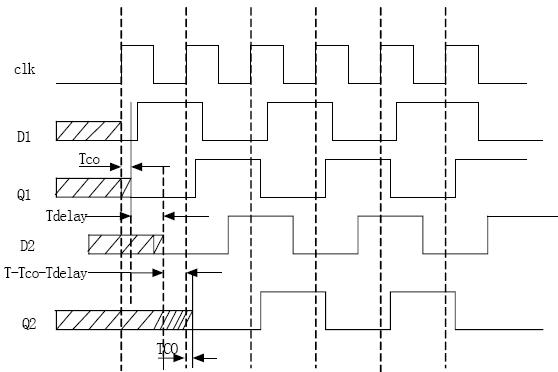Figure 4 Shows the timing chart that meets the requirements

From the figure 4 we can see:

T-Tco-Tdelay>T3

That is

Tdelay< T-Tco-T3

During the setup time D2, the signal can reach D2 through the combinational logic D1, i.e. the data is already in Tsup before the second CLK arrive.

Then it meets the requirement of setup time, where T as the clock period, the second flip-flop can pick up D2 on the rising edge of the second clock in this case.

{D1 => setup time => hold time => trigger data output delay => combinational logic delay => D2 => ...}

If the delay time of the combinational logic is too large

T-Tco-Tdelay < T3 (Tcox<D2 setup time)

Then it will not meet the requirements. The second trigger will pick up an unstable state on the rising edge of the second clock, as shown in Figure 5, then the circuit will not work properly.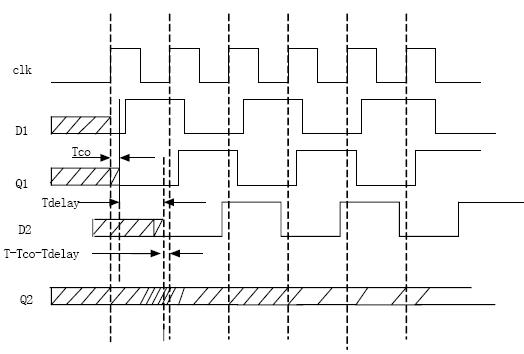Figure 5 The delay time of the combinational logic is too large to meet the requirements

So you can derive

T - the Tco - T2max > = T3

This is the setup time for D2.

From the timing diagram above, it also can be seen that the setup time and hold time of D2 are not related to the setup and hold time of D1, except the combinational logic in front of D2 and the data transmission delay of D1. This is also a Very important conclusion, which shows that the delay has no additive effect.

However, if there is a delay in the clock instead, the hold time must be considered in this case, together with the setup time. Most clocks with large delays are designed using asynchronous clocks, which is difficult to guarantee the data synchronization, so it is rarely used in actual designs. At this point, if the setup time and hold time all meet the requirements, you will see the output timing as shown in Figure 6.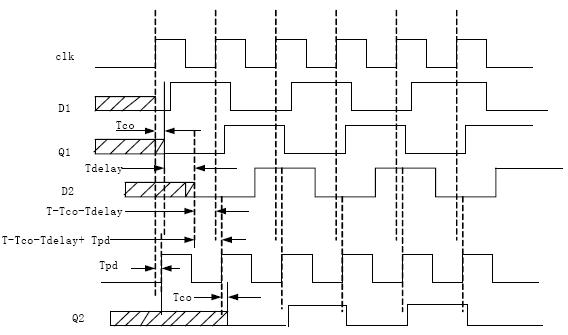Figure 6. Clock has a delay but meets the timing

It can be easily seen from figure 5 that the Tpd is relaxed for the setup time, so the setup time of D2 must meet the requirements:

Tpd+T-Tco-T2max>=T3

(T3 is the setup time of D2, T2max is the maximum delay of  combinatorial logic, Tpd is the clock delay)

As shown in the FIG. 6, since the sum of setup time and hold time is a stable clock period (T), if the clock has a delay and the data delay is small, then the setup time will increase inevitably,  and the decrease of hold time goes with it. If it is reduced to not meet the requirement of hold time D2 , the correct data cannot be collected.

That is

T-(Tpd-Tco-T2min)

T-(Tpd+T-Tco-T2min)>=T4

i.e. Tco+T2min-Tpd>=T4 (D2 hold time )

From the formula above we could also figure out that if Tpd = 0, that is to say the delay of the clock is 0, then the same requirements goes with Tco + T2min> T4, however in practical applications the delay of T2 i.e. the delay of line is much larger than the trigger's hold time T4, it becomes not necessary to take the hold time into consideration.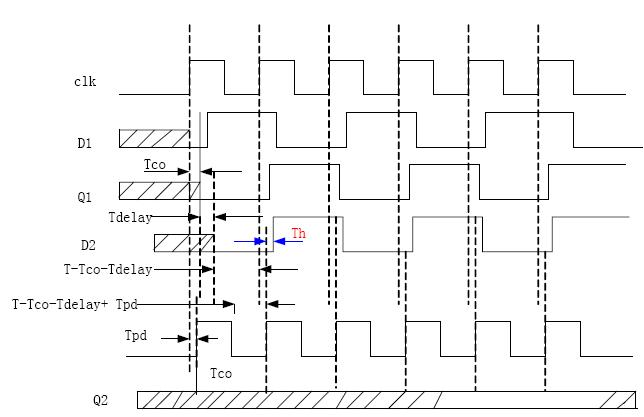Figure 7 The clock has a delay and the hold time does not meet requirements

In summary, if you do not consider the delay of the clock, the only thing you need to care about is the setup time, or the hold time instead.

Then let us think about in FPGA design, how to increase the working clock in the synchronous system.

Analysis

Ⅳ. How to increase the clock working frequency

From the above analysis, we can see that the requirements of setup time T3 for the D2 in the synchronization system is as follows:

T-Tco-T2max>=T3

So it is easy to derive:

T>=T3+Tco+T2max

where T3 is the setup time Tset of D2, and T2 is the delay time of the combinational logic.

In a design, T3 and Tco are both fixed values determined by the device, the only factor that we could control is the input delay of the combination logic T2. Therefore, by reducing T2 as much as possible, the clock working frequency can be increased. In order to achieve the reduction of T2 in the design, there are different comprehensive methods we can use.

1. Changing the line type for circuit wiring

Altera devices, for example, there are many bars in the quartus timing closure floorplan, so we can slice and dice them into rows and columns:

Each bar represents 1 LAB, each LAB has 8 or 10 LEs in.

The relationship of their routing delay is as follows:

the same LAB (fastest) < the same row and column < different row and column. We could add appropriate constraints to the synthesizer (this should be given appropriate, generally 5% margin adding, for example, if the circuit works at 100Mhz, then adding constraints to 105Mhz is sufficient, because the excessive constraint could do a bad effect instead, and greatly increases the integration time) to make the relevant logic circuit wiring be placed as close as possible, thereby reducing the routing delay.

2. Splitting the combinational logic

Since the general synchronous circuits are more than a?single?stage latch (as shown in Figure 9), and to make the circuit stable, the clock period must meet the maximum delay requirement, and the maximum?delay of the longest path can be shortened before the operating frequency of the circuit be increased.

As shown in Figure 8, we can decompose the larger combinatorial logic into smaller blocks and insert flip-flops in the middle, which can increase the operating frequency of the circuit. This is also the basic principle of the so-called "pipelining" technology.

For the upper part of Figure 9, its clock frequency is subject to the delay of the second larger combinational logic. By appropriately distributing the combinational logic, excessive delay between the two flip-flops can be avoided and speed bottlenecks can be eliminated.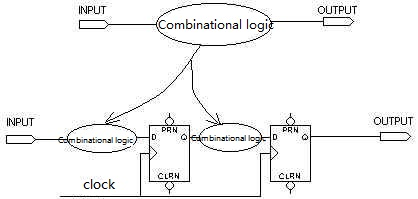Figure 8 Splitting combination logic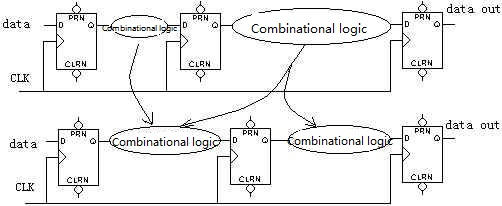Figure 9 Transferring Combination Logic

How to split the combinatorial logic in design, the better method should be accumulated in practice, but some good design ideas and methods also need to be mastered. We know that at present most of the FPGAs based on 4-input LUTs, if an output criteria corresponding is more than four inputs, then the multiple LUT cascade will be needed, thus introducing the delay of one-stage combinational logic. That is we want to reduce the number of combinational logic, the logic is nothing more than to make the input conditions as few as possible, so that less multiple LUT cascade need to be use, thereby reducing the time delay caused by combinational logic.

The pipelining that we usually hear is a way to increase the operating frequency by splitting a large combinational logic (in the middle of which a singer or multiple stages of D flip-flops are inserted, thereby reducing the number of combinatorial logic between registers) to a smaller one.

For example, a 32-bit counter, with a very long carry chain, will inevitably reduce the operating frequency, so we can split it into a 4-bit and a 8-bit one, whenever the 4-bit counter counts to 15 and triggers an 8-bit one, which enable the counter to be split and increases the operating frequency.

Just as the same, large counters are generally moved out of the state machine, because if they, with usually more than 4 inputs, are used as state transition criteria with other conditions, they will increase the multiple LUT cascade, and then increasing the combination logic.

Taking a 6-input counter as an example, we wanted to make a state transition after the counter counted to 111100, now because we put the counter out of the state machine, when it counts to 111011, a signal of "enable" is generated and then trigger the state transition, which obviously reduces the combinatorial logic.

3. The composition of the state machine

The state machine generally contains three modules:

• An output module

• A module that determines what the next state is

• A module that saves the current state

The logic used to form these three modules is also different. The output module usually contains both combinatorial logic and sequential logic; the module that determines the next state is usually composed of combinatorial logic; and the module that saves the current state is usually composed of sequential logic.

The relationship between these three modules is shown in Figure 10.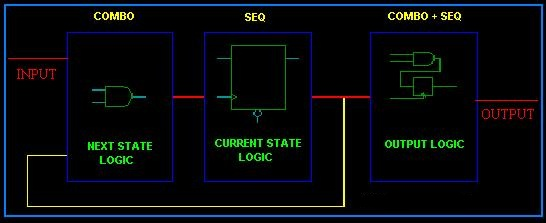Figure 10 The composition of the state machine

Ⅴ. An example showing a good method for state machine design

That is why when writing the state machine, the state machine is always divided into three parts according to these three modules. The following example shows a good method of state machine design:

-----------------------------------------------------*/

module arbiter2 (

clock , // clock

reset , // Active high, syn reset

req_0 , // Request 0

req_1 , // Request 1

gnt_0 ,

gnt_1

);

//-------------Input Ports-----------------------------

input    clock ;

input    reset ;

input    req_0 ;

input    req_1 ;

//-------------Output Ports----------------------------

output    gnt_0 ;

output    gnt_1 ;

//-------------Input ports Data Type-------------------

wire    clock ;

wire    reset ;

wire    req_0 ;

wire    req_1 ;

//-------------Output Ports Data Type------------------

reg        gnt_0 ;

reg        gnt_1 ;

//-------------Internal Constants--------------------------

parameter     SIZE = 3 ;

parameter     IDLE = 3'b001 ,

GNT0 = 3'b010 ,

GNT1 = 3'b100 ;

//-------------Internal Variables---------------------------

reg        [SIZE-1:0] state ;        // Seq part of the FSM

wire    [SIZE-1:0] next_state ;    // combo part of FSM

//----------Code startes Here------------------------

assign    next_state = fsm_function(req_0, req_1);

//------------fsm_function--------------//

function [SIZE-1:0] fsm_function;

input     req_0;    //parameter

input     req_1;    //parameter

begin

case(state)

IDLE :

if (req_0 == 1'b1)

fsm_function = GNT0;

else if (req_1 == 1'b1)

fsm_function = GNT1;

else

fsm_function = IDLE;

GNT0 :

if (req_0 == 1'b1)

fsm_function = GNT0;

else

fsm_function = IDLE;

GNT1 :

if (req_1 == 1'b1)

fsm_function = GNT1;

else

fsm_function =IDLE;

default : fsm_function = IDLE;

endcase

end

endfunction

always@(posedge clock)

begin

if (reset == 1'b1)

state <= IDLE;

else

state <= next_state;

end

//----------Output Logic-----------------------------

always @ (posedge clock)

begin

if (reset == 1'b1)

begin

gnt_0 <= #1 1'b0;

gnt_1 <= #1 1'b0;

end

else

begin

case(state)

IDLE :

begin

gnt_0 <= #1 1'b0;

gnt_1 <= #1 1'b0;

end

GNT0 :

begin

gnt_0 <= #1 1'b1;

gnt_1 <= #1 1'b0;

end

GNT1 :

begin

gnt_0 <= #1 1'b0;

gnt_1 <= #1 1'b1;

end

default :

begin

gnt_0 <= #1 1'b0;

gnt_1 <= #1 1'b0;

end

endcase

end

end // End Of Block OUTPUT_

Endmodule

Ⅵ. The introduction of state machine

State machines are usually written in three segments to avoid excessive combinational logic.

All we mentioned above shows how we could use the way of pipelining to split the combinational logic, but in some cases it is difficult for us to do that, and then what should we do?

The state machine is such an example that we cannot add assembly line in the state decoding combinational logic. If there is a design of state machine with dozens of states, there is no doubt that its state decoding logic will be very large and this will be the critical path in the design. So what should we do?

Just the same way, reducing the combinatorial logic. We can analyze the output of the state, reclassify and redefine them into a group of small state machines. By selecting the input (case statement) and triggering the corresponding small state machine, we can achieve a large state machine splitting into several small state machines. In the ATA6 specification (hard disk standard), there are about 20 kinds of input commands, and each piece of command corresponds to a variety of states. It is unthinkable to do it with a large state machine (nesting), however in the contrary, if you use the case statement to decode the command and trigger the corresponding state machine, in this way the module can run very fast.

The key to increasing the operating frequency is to reduce the time delay from register to register, and the most effective method for reduction is to avoid large combinational logic, that is, to try to meet the four-input condition, reducing the number of LUT cascades, that’s mean that we could increase the working frequency by adding constraints, using a way of pipelining and splitting states.

Ⅶ. What we should pay attention when designing the clock in FPGA

1. 1.Try to use only one clock in a module, and a module here means a module or an entity. In the design of multi-clock domain, it is better to have an extra special module for the isolation of clock domain. This allows the synthesizer to get a better results.

2. 2. Unless it is a low-power design, otherwise do not use the gated clock (gllobal Clock buffer such as IBUFG within FPGA) to control the input of clock edge of flip-flop, but use combinational logic and other timing logic (such as frequency divider) to generate signals used as the input of clock edge of flip-flop---all this is to reduce the instability of the design.

3. 3. Do not use the signals divided by counter as the clock of other modules, but  with the help of clock enable(CE). Otherwise, this clock-like manner is extremely unfavorable to the reliability of the design, and greatly increases the complexity of the static timing analysis .

Ⅷ. Synchronization Between Different Clock Domains

If two modules in a design using two respective operating clock, then at their interfaces there would emerge a phenomenon which called as Asynchronous Patterns. In order to ensure data correct processed, the two modules must be synchronized.

There are usually two cases of different clock domains here (discrete clock source):

1. 1. the frequency of two clocks is different;

2. 2. the two clocks share a same frequency, but they are actually two separate clocks with no relation to the phase.

Just as shown in the following two figures: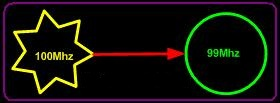Figure 11 The frequencies of two clocks are completely differentFigure 12 The frequencies of the two clocks are the same, but the phases are irrelevant

The data transmission between two clock domains usually adopts different synchronization methods according to different bit widths.

1. Synchronization between single bits and each pulse transmitted has at least 1 cycle width

This kind of synchronization is mainly used for the synchronization of some control signals. As shown in Figure 13 below: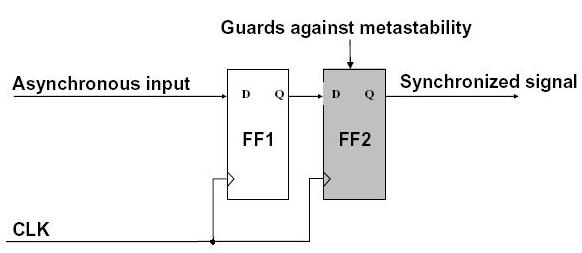Figure 13 One bit synchronizer design

The following points are required to be explained for this synchronization:

(1) synchronous circuit of figure 12 is actually called "one bit synchronizer", it can only be used for one bit asynchronous signal which must be wider than that of the Current stage’s clock, otherwise it may be unable to adopt this asynchronous signal.

(2) why is the circuit in figure 13 can only be used in one bit asynchronous signals?

When two or more asynchronous signals (control or address) simultaneously get into the current time domain and take control the circuit of current time domain, problems arise if these signals are all synchronized using the same circuit in FIG. 13. Skews has arisen between two or more asynchronous signals (control or address) due to connection delays or other delays, and then the skew is greatly enlarged via the synchronizer in Figure 13 when getting into the current time domain, or competition may caused and finally leading to an error in the time domain circuit.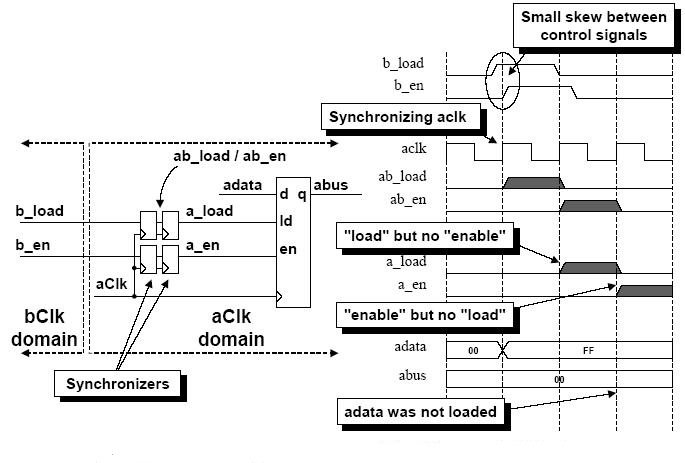Figure 14 Problem-passing multiple control signals between clock domains

If the asynchronous data bus is to enter the current time domain, the circuit in Figure 13 cannot be used either, because data change very randomly and the width of 0 or 1 has nothing to do with the clock pulse of the current time domain, so the circuit in Figure 13 may be unable to adopt the correct data.

(3) Please note that the second trigger is not used for avoiding the occurrence of "metastable state", on the contrary, it can prevent the transmission of metastable state. In other words, once the first flip-flop becomes metastable (possibly), due to the second flip-flop, the metastability will not be transmitted to the circuit following.

(4) The first-stage trigger has a metastable state, which means it will require a recovery time to stabilize again, or it is also called Withdrawal from metastable state. The recovery time plus the establishment time of the second-stage flip-flop (say more precisely, maybe also minus the clock skew) is less than or equal to the clock period, which can be easily satisfied. This is means thees two stages of flip-flop should be put together as close as possible, without any combinatorial logic between them or excessive skews to the clock, and then the second-stage flip-flop can adopt data stably and preventing the transmission of metastable state.

(5) FF1 is the sampling output of FF2, so of course, what is output by FF1 is  what output by FF2, everything is the same except one cycle of delay. Note that “meta-stableit” means that once the data of FF1 enters, its electrical level would be indefinite and maybe incorrect. So although this method can prevent transmission of metastable state, it does not guarantee the data after the two-stage flip-flop is correct. Therefore, this kind of circuit always has a certain amount of fault-tolerance. This applies only to a some error-insensitive cases, but for other sensitive circuits, dual-port RAM or FIFO are better choices.

2. The input pulse could be less than a synchronous circuit under a clock cycle width

How is that possible? Has it not less than the original clock? For this case, the Feedback shown in Figure 15 below may usually be taken into consideration. The analysis of this circuit is as follows:

Assume that the input data is high level, because the first flip-flop FF1 is high-level cleared, then all outputs should also be high and correctly adopted. On the other hand, if the input is low-level, data of FF1 would be forced to clear and the output level is zero, which ensures the correctness of the output.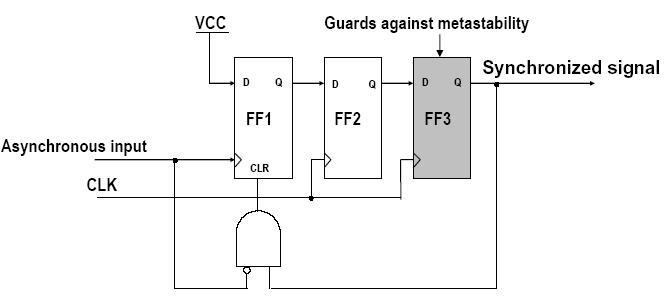Figure 15 Synchronous circuit--input pulse may be less than one clock cycle width

Book Suggestion

Building Embedded Systems: Programmable Hardware 1st ed. Edition

This is a book for embedded-system engineers and intermediate electronics enthusiasts who are seeking tighter integration between software and hardware. Those who favor the System on a Programmable Chip (SOPC) approach will in particular benefit from this book. Students in both Electrical Engineering and Computer Science can also benefit from this book and the real-life industry practice it provides.

--Changyi Gu

Digital Integrated Circuit Design Using Verilog and Systemverilog 1st Edition, Kindle Edition

For those with a basic understanding of digital design, this book teaches the essential skills to design digital integrated circuits using Verilog and the relevant extensions of SystemVerilog. In addition to covering the syntax of Verilog and SystemVerilog, the author provides an appreciation of design challenges and solutions for producing working circuits.

--Ronald W. Mehler

Power Converters with Digital Filter Feedback Control 1st Edition, Kindle Edition

This book builds a bridge for moving a power converter with conventional analog feedback to one with modern digital filter control and enlists the state space averaging technique to identify the core control function in analytical, close form in s-domain (Laplace). It is a useful reference for all professionals and electrical engineers engaged in electrical power equipment/systems design, integration, and management.

--Keng C. Wu

Relevant information "Discussion on the influencing factors of clock in FPGA design"

About the article "Discussion on the influencing factors of clock in FPGA design", If you have better ideas, don't hesitate to  write your thoughts in the following comment area.

You also can find more articles about electronic semiconductor through Google search engine, or refer to the following related articles.HOW TO BUY ELECTRONIC COMPONENTS
Search
Inquiry
Order
Track

Delivery
FedEx
UPS
DHL
TNT

Payment Terms
By PayPal
By Credit Card
By Wire Transfer
By Western Union

After-sales Service
Quality Control
Guarantee
Return & Replacement